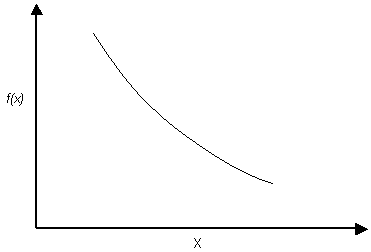Expert Modelling: Hands-on help from Rocscience Engineers. Register Here

# Exponential Distribution

The Exponential probability density function is available in CPillar.

Exponential probability density functionNOTES:

• The range of values must always be positive for an Exponential distribution, therefore it should not be used for variables which may include negative values.
• The mean is always equal to the standard deviation for an Exponential distribution. This is a property of the Exponential distribution, and cannot be altered by the user.
• Like the Normal distribution, the Exponential distribution can be truncated by entering the desired minimum and maximum values (the basic Exponential distribution varies from zero to infinity).
• The Exponential distribution is sometimes used to define events, such as the occurrence of earthquakes or rock bursts, or quantities such as the length of joints in a rock mass.